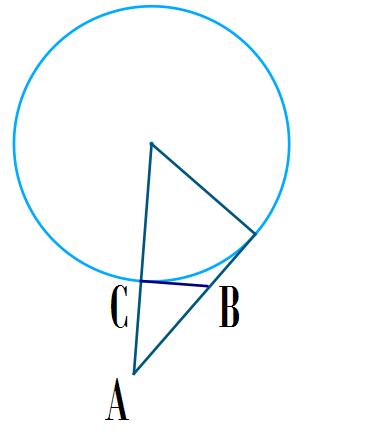The diagrams shows a circle with radius $r$ with a straight line tangent to the circle, the straight line passes through the point $A$ and $B$. Given that $C$ is on the point of $C$. And the distances $\overline{AC} = 4, \overline{BC} = 3, \overline{AB} = 5$. Find the radius of the circle.